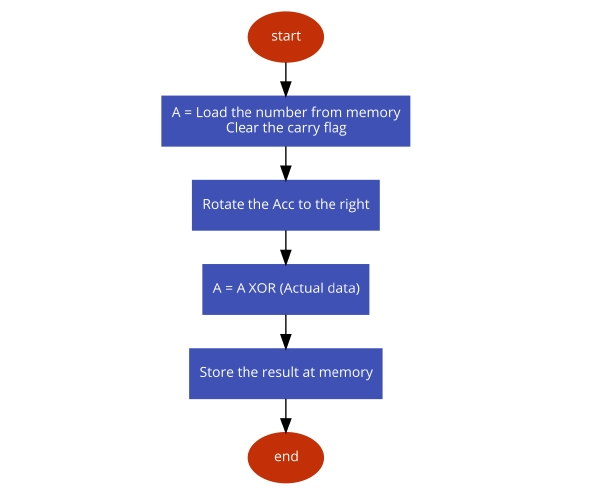# 8085 program to convert binary numbers to gray

In this program we will see how to find the gray code from an 8-bit number.

### Problem Statement

Write 8085 Assembly language program to convert an 8-bit number stored at 8000H to its equivalent gray code. The result will be stored at 8050H.

### Discussion

In this program we are converting binary to gray code. The procedure is simple. At first we have to shift the content to the right, then perform XOR operation with the sifted content and the actual content. Thus we will get the gray code. For an example if the number is ABH, then the binary value will be (1010 1011), after shifting the value will be (0101 0101) = 55H, now by XORing ABH and 55H, the result will be (1111 1110) = FEH

first input

......
8000AB
......

second input

......
800077
......

third input

......
8000CD
......

### Flow Diagram### Program

F00021, 00, 80LXI H,8000HPoint to the source address
F0037EMOV A, MTake the number from memory to Acc
F00437STCSet carry flag
F0053FCMCComplement carry flag
F0061FRARRotate right Acc content
F007AEXRA M   XOR memory content with A
F00832, 50, 80STA 8050H   Store the gray code
F00B76HLTTerminate the program

first input

......
8050FE
......

second input